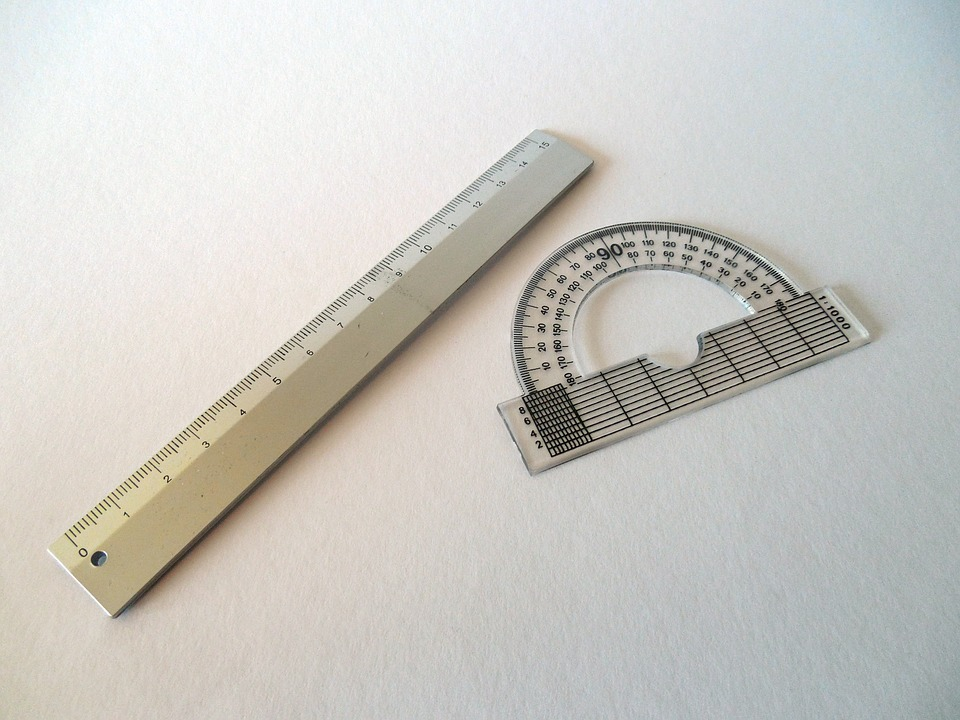# How to Use a Protractor

A protractor is used to construct and to measure angles of specific measures. They are used to create the angles and to draw straight lines. In a school setting, the protractor is used in the study of geometry. There are different steps that are followed when carrying out each of these tasks. These are:

To measure angles

• Locate the center hole on the edge of the protractor.

• Place the hole in the center of the protractor over the central point of the angle being measured.

• Ensure that the angle is lined up on the zero point at the end of the protractor. Ensure that it is straight. Make sure that the other side of the angle is seen with ease and should be lined up along the numbers on the protractor.

• Locate the point of intersection of the curved edge and the protractor to determine the size of the angle.

• Read the degree indicated on the protractor at the point of intersection and record it as the size of the angle in degrees.

To draw angles

In geometry one is may also be required to draw some angles using the protractor. In this case the following steps should be followed:

• Draw a straight line on the page using the straight edge of the protractor. The line drawn will be the straight part of the angle drawn.

• Place the protractor at the mid point of the line by placing dots on the two ends of the line drawn. Use the two dots made as the starting points. Make a dot at the mid point found and place the dot underneath the point zero on the protractor.

• Place a dot beneath the angle that is being drawn from the reading on the protractor. For instance, where the angle being drawn is 60 degrees, position the protractor over the first line drawn and mark the point where the angle falls on the curved edge of the protractor.

• Draw a line using the straight side of the protractor between the points marked zero and join it to the dot marked alongside the angle being drawn.

• This will form the angle desired.

To draw lines

The straight edge of the protractor is used often to draw lines especially when marking and drawing and angle and when measuring the size of angles. The ruler acts as a guide in this case. The protractor is used to draw dots at the points where one needs to have their angles and to join the dots marked in a straight line. In addition, it helps in the determination of how short or long an angle will be. Using a protractor in measuring angles and drawing lines is a simple task and following the above described do-it-yourself procedure makes it even easier.